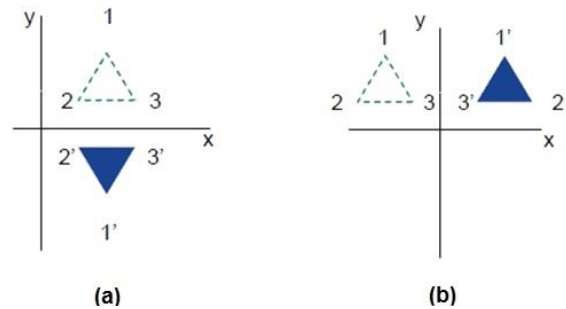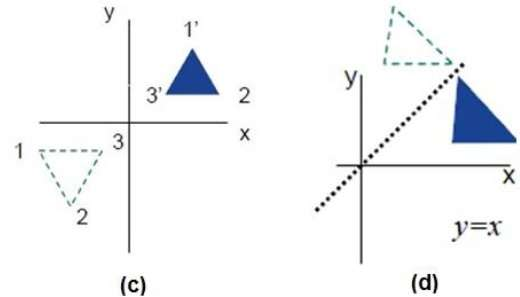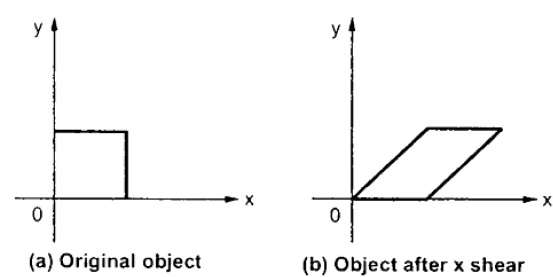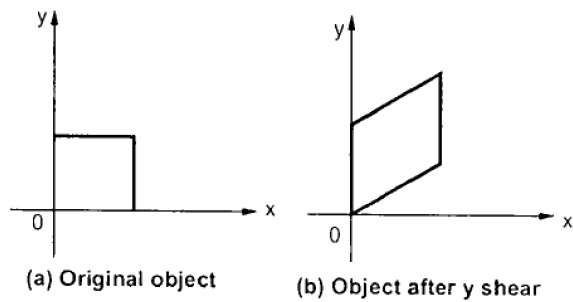# Reflection and Shearing – Two-dimensional Geometric Transformations

## Reflection

Reflection is the mirror image of original object. In other words, we can say that it is a rotation operation with 180°. In reflection transformation, the size of the object does not change.

The following figures show reflections with respect to X and Y axes, and about the origin respectively.## Shear

A transformation that slants the shape of an object is called the shear transformation. There are two shear transformations X-Shear and Y-Shear. One shifts X coordinates values and other shifts Y coordinate values. However; in both the cases only one coordinate changes its coordinates and other preserves its values. Shearing is also termed as Skewing.

### X-Shear

The X-Shear preserves the Y coordinate and changes are made to X coordinates, which causes the vertical lines to tilt right or left as shown in below figure.The transformation matrix for X-Shear can be represented as −

Xsh=⎡⎣⎢100shx10001⎤⎦⎥Xsh=[1shx0010001]

Y’ = Y + Shy . X

X’ = X

### Y-Shear

The Y-Shear preserves the X coordinates and changes the Y coordinates which causes the horizontal lines to transform into lines which slopes up or down as shown in the following figure.The Y-Shear can be represented in matrix from as −

Ysh⎡⎣⎢1shy0010001⎤⎦⎥Ysh[100shy10001]

X’ = X + Shx . Y

Y’ = Y